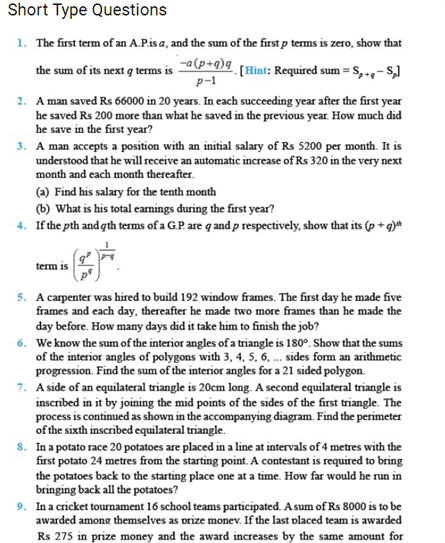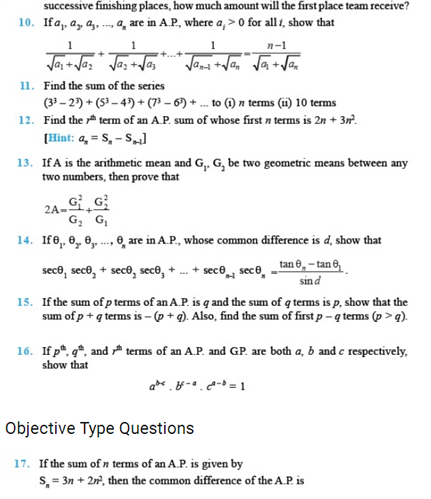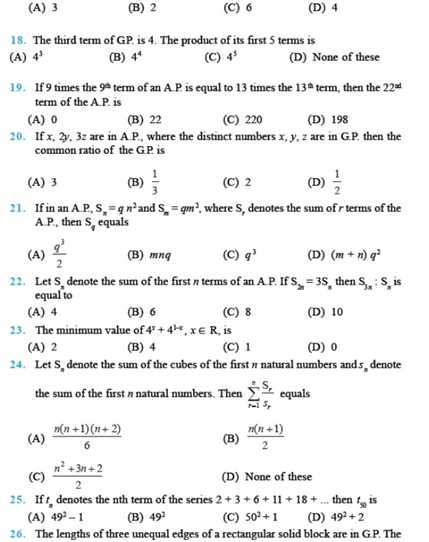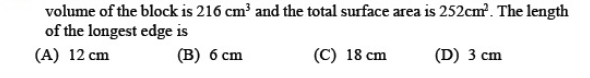# Chapter 9 - Sequences and Series

Some of the most important Sequence and Series Class 11 questions i.e chapter 9 are given here. These chapter 9 class 11 maths questions cover several short answer type questions, long answer type questions and HOTS questions that are crucial for CBSE class 11 exams. Here, some of the important sequence and series questions from NCERT class 11 are also included.

Also, Check:

Class 11 Sequence and Series Notes

Arithmetic Progression

Geometric Progression

## Sequence and Series Important Questions For Class 11 (Chapter 9)

These class 11 Sequence and Series questions are categorized into short answer type questions and long answer type questions. These questions cover various concepts which will help class 11 students to develop problem-solving skills for the exam.

### Sequence  and Series Extra Questions

1. Prove that the sequence tn = 3n + 5 is an Arithmetic Progression. Find its common difference.
2. Find the 10th common term between the AP
• 3+7+ 11+….. &
• 1 + 6 + 11 +16
3. Is 309 a term of the Ap 11, 17, 23….
4. The sum of 3 numbers of an arithmetic progression is 24 and their product is 44. Find the three numbers.
5. If the sum of first n terms of a progression is a quadratic expression in n, show that it is an arithmetic progression.
6. If the AM between the ath and bth terms of an AP be equal to the AM between the cth and dth term of it then prove that  a + b = c+ d
7. Find three numbers whose product is 1728 and sum is 38.
8. What will Rs 5000 amount to in 10 years, compounded annually at 10 % per annum?
9. Find two positive numbers m and n whose AM and GM are 34 and 16 respectively.
10. Find the sum of the series – 1 + 5 + 12 +….to n terms.

### Sequence and Series NCERT Questions#### 1 Comment

1. Shivesh kumar

Sequence and series questions